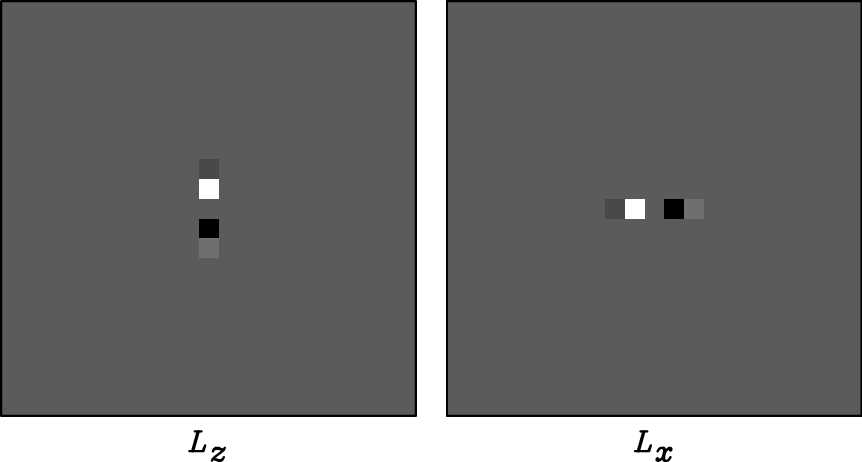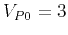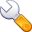Elastic wave-mode separation for VTI mediaNext: Operator size and compactness Up: Operator properties Previous: Operator properties

## Operator orders

As I have showed in the previous section, the isotropic separation operators (divergence and curl) in equations 4 and 5 are exact in theanddomains. The exact derivative operators are infinitely long series in the discretized space domain. In practice, when evaluating the derivatives numerically, one needs to take some approximations to make the operators short and computationally efficient. Usually, difference operators are evaluated at different orders of accuracy. The higher order the approximation is, the more accurate and longer the operator becomes. For example, theorder operator has coefficients, and the more accurateorder operator has coefficients(Fornberg and Ghrist, 1999).

In the wavenumber domain, for isotropic media, as shown by the black line in Figure 2(b), the exact difference operator is. Appendix A shows thedomain equivalents of the,,, andorder finite difference operators, and they are plotted in Figure 2(b). The higher order operators have responses closer to the exact operator(black line). To obtain vertical and horizontal derivatives of different orders of accuracy, I weight the polarization vectorcomponentsandby the weights shown in Figure 2(c). For VTI media, similarly, I weight the anisotropic polarization vectorcomponentsandby these same weights. The weighted vectors are then transformed back to space domain to obtain the anisotropic stencils.operator
Figure 2.
Comparison of derivative operators of different orders of accuracy (,,, andorders in space, as well as the approximation applied in Dellinger and Etgen (1990)-cosine taper) in both (a) thedomain and (b) thedomain. (c) Weights to apply to the components of the polarization vectors.iop2,mop2,iop4,mop4,iop6,mop6,iop8,mop8
Figure 3.,,, andorder derivative operators for an isotropic medium (km/s andkm/s) and a VTI medium (km/s,km/s,and). The left column includes isotropic operators, and the right column includes anisotropic operators. From top to bottom are operators with increasing orders of accuracy.Elastic wave-mode separation for VTI mediaNext: Operator size and compactness Up: Operator properties Previous: Operator properties

2013-08-29